Example Questions

1 2 3 5 Next →

Example Question #1 : Inequalities

What is the solution set of the inequality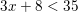?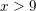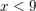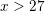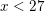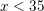Explanation:

We simplify this inequality similarly to how we would simplify an equation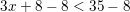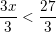ThusExample Question #6 : Inequalities

What is a solution set of the inequality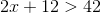?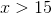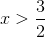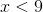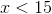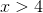Explanation:

In order to find the solution set, we solveas we would an equation: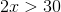Therefore, the solution set is any value of.

Example Question #1 : How To Find The Solution To An Inequality With Division

Which of the following could be a value of, given the following inequality?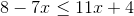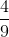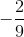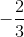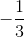Explanation:

The inequality that is presented in the problem is:Start by moving your variables to one side of the inequality and all other numbers to the other side: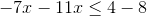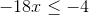Divide both sides of the equation by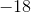. Remember to flip the direction of the inequality's sign since you are dividing by a negative number!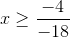Reduce: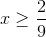The only answer choice with a value greater than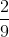is.

Example Question #42 : Inequalities

If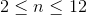and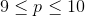, which of the following gives the set of possible values of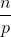?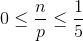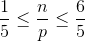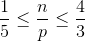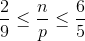Explanation:

To get the lowest value, you need the lowest numerator and the highest denominator.  That would be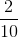or reduced to be.  For the highest value, you need the highest numerator and the lowest denominator.  That would be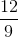or.

Example Question #43 : Inequalities

Give the solution set of this inequality: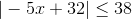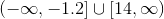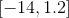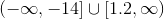The inequality has no solution.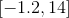Explanation:

The inequalitycan be rewritten as the three-part inequality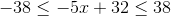Isolate thein the middle expression by performing the same operations in all three expressions. Subtract 32 from each expression: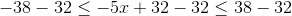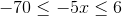Divide each expression by, switching the direction of the inequality symbols: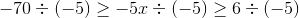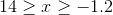This can be rewritten in interval notation as.

Example Question #44 : Inequalities

Give the solution set of this inequality: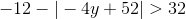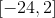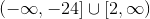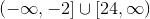The inequality has no solution.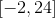The inequality has no solution.

Explanation:

In an absolute value inequality, the absolute value expression must be isolated first, as follows: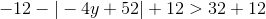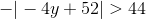Multiplying both sides by, and switching the inequality symbol due to multiplication by a negative number: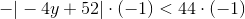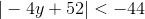We do not need to go further. An absolute value expression must always be greater than or equal to 0; it is impossible for the expression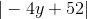to be less than any negative number. The inequality has no solution.

1 2 3 5 Next →